# High School Math : Finding Domain and Range

## Example Questions

### Example Question #1 : Relations And Functions

What is the domain of the function below: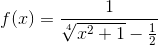Possible Answers: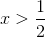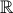Correct answer:Explanation:

The domain is defined as the set of possible values for the x variable. In order to find the impossible values of x, we should:

a) Set the equation under the radical equal to zero and look for probable x values that make the expression inside the radical negative: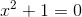There is no real value for x that will fit this equation, because any real value square is a positive number i.e. cannot be a negative number.

b) Set the denominator of the fractional function equal to zero and look for probable x values: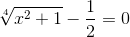Now we can solve the equation for x: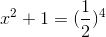There is no real value for x that will fit this equation.

The radical is always positive and denominator is never equal to zero, so the f(x) is defined for all real values of x. That means the set of all real numbers is the domain of the f(x) and the correct answer is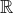.

Alternative solution for the second part of the solution:

After figuring out that the expression under the radical is always positive (part a), we can solve the radical and therefore denominator for the least possible value (minimum value). Setting the x value equal to zero will give the minimum possible value for the denominator.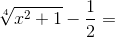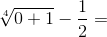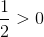That means the denominator will always be a positive value greater than 1/2; thus it cannot be equal to zero by setting any real value for x. Therefore the set of all real numbers is the domain of the f(x).

### Example Question #1 : Function Properties

What is the domain of the function below?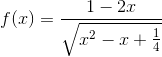Possible Answers: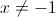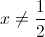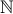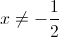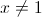Correct answer:Explanation:

The domain is defined as the set of all values of x for which the function is defined i.e. has a real result. The square root of a negative number isn't defined, so we should find the intervals where that occurs: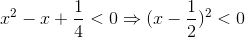The square of any number is positive, so we can't eliminate any x-values yet.

If the denominator is zero, the expression will also be undefined.

Find the x-values which would make the denominator 0: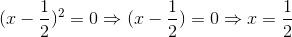Therefore, the domain is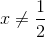.

### Example Question #1 : Finding Domain And Range

What is the domain of the function below: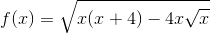Possible Answers: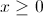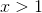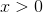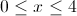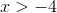Correct answer:Explanation:

The domain is defined as the set of all possible values of the independent variable. First,must be greater than or equal to zero, because if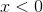, then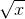will be undefined. In addition, the total expression under the radical, i.e.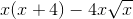must be greater than or equal to zero: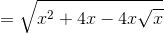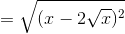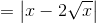That means that the expression under the radical is always positive and thereforeis defined. Hence, the domain of the functionis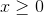.

### All High School Math Resources